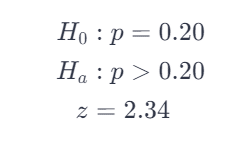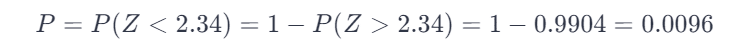# Suppose We Are Testing Ho: P=.20 Vs Ha: P Does Not Equal .20 And Ts=2.34. What Is The P-value?

We thoroughly check each answer to a question to provide you with the most correct answers. Found a mistake? Let us know about it through the REPORT button at the bottom of the page.

Suppose we are testing Ho: p=.20 vs Ha: p>.20 and TS=2.34. What is the p-value?

Given (TS = test statistic z)The P-value is the probability of obtaining the value of the test statistic, or a value more extreme when the null hypothesis is true. Determine the P-value using the normal probability table in the appendix.Note: Command for a Ti83/84-calculator is normal cdf(2.34,qE99) or 1E99 replaced by some other very large value.